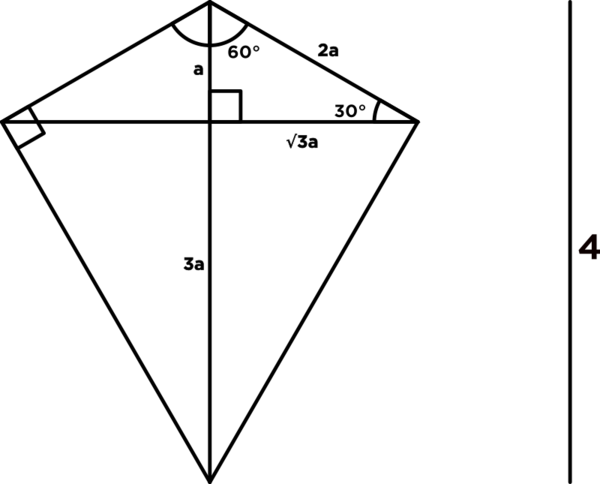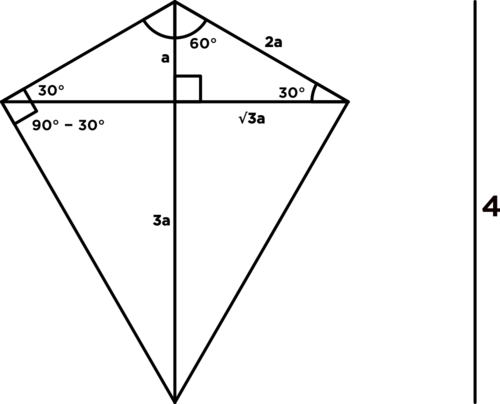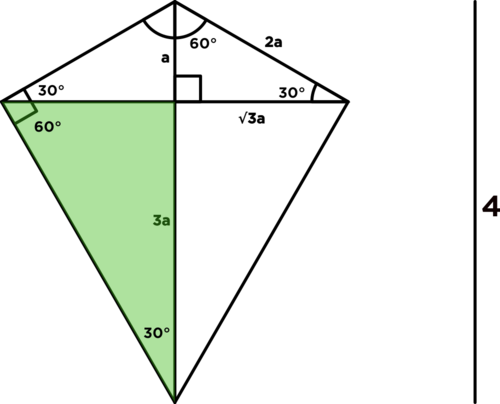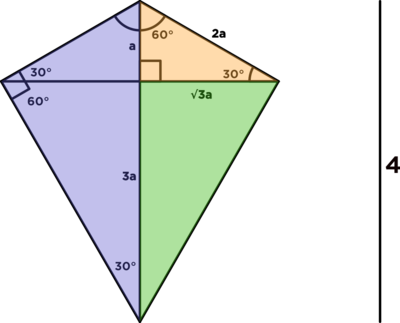# It says "There is a large 30°-60°-90° right triangle whose hypotenuse is the vertical line in the figure." Which vertical line are you talking about?

• Module 2 Week 1 Day 4 Your Turn Part 2

It says "There is a large 30°-60°-90° right triangle whose hypotenuse is the vertical line in the figure." Which vertical line are you talking about?

• @divinedolphin Thanks for asking! You are right, this is slightly ambiguous, because the diagram is rife with lines.The large \(30^{\circ}-60^{\circ}-90^{\circ}\) right triangle has a hypotenuse which is the vertical line labeled with a "4."This large \(30^{\circ}-60^{\circ}-90^{\circ}\) right triangle makes up half of the kite.

This diagram has a lot of cool analogous angles which seem to come out one by one. You can see this by starting with the right angle on the left (which is the mirror image of the right). We've got a \(30^{\circ}\) degree angle from symmetry on the right, which means the lower angle is the complement of this, \(90^{\circ} - 30^{\circ},\) which equals \(60^{\circ}.\)Wait a second, this \(60^{\circ}\) angle is inside a right triangle (colored green), so its third angle is then complementary to \(60^{\circ}\) and must be equal to \(90^{\circ} - 60^{\circ} = 30^{\circ},\) so the green triangle is also a \(30^{\circ}-60^{\circ}-90^{\circ}\) triangle!Lo and behold, there are three differently-sized \(30^{\circ}-60^{\circ}-90^{\circ}\) right triangles in this diagram:• @debbie What a bunch of 30∘−60∘-90∘ right triangles!